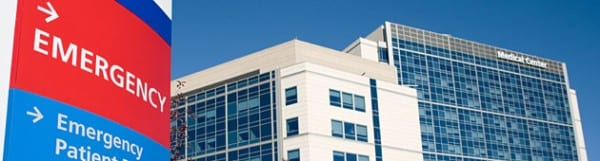Select Page

# Statistics on Independent Physicians

Curated by Paul Helmick

I’m a Technology CEO and Experienced Entrepreneur. I love helping people use technology to grow their business.Figures show that while the physician workforce has expanded by roughly 110,000 physicians since 2000, the number of independent providers has dropped by at least 18 percent in that same timeframe. Physicians were defined as independent if they owned at least part of a practice.### 2000

• Practicing physicians: 682,470
• Percent independent: 57 percent
• Estimated number of independent physicians: 389,008

### 2001

• Practicing physicians: 690,369
• Percent independent: 55 percent
• Estimated number of independent physicians: 381,228

### 2002

• Practicing physicians: 698,359
• Percent independent: 53 percent
• Estimated number of independent physicians: 373,603

### 2003

• Practicing physicians: 706,441
• Percent independent: 52 percent
• Estimated number of independent physicians: 366,131

### 2004

• Practicing physicians: 714,617
• Percent independent: 50 percent
• Estimated number of independent physicians: 358,809

### 2005

• Practicing physicians: 722,888
• Percent independent: 49 percent
• Estimated number of independent physicians: 351,632

### 2006

• Practicing physicians: 731,254
• Percent independent: 47 percent
• Estimated number of independent physicians: 344,600

### 2007

• Practicing physicians: 739,718
• Percent independent: 46 percent
• Estimated number of independent physicians: 337,708

### 2008

• Practicing physicians: 748,279
• Percent independent: 44 percent
• Estimated number of independent physicians: 330,954

### 2009

• Practicing physicians: 756,939
• Percent independent: 43 percent
• Estimated number of independent physicians: 324,334

### 2010

• Practicing physicians: 765,700
• Percent independent: 42 percent
• Estimated number of independent physicians: 317,848

### 2011

• Practicing physicians: 774,562
• Percent independent: 41 percent
• Estimated number of independent physicians: 313,703

### 2012

• Practicing physicians: 783,526
• Percent independent: 39 percent
• Estimated number of independent physicians: 305,575

### 2013 (Projected)

• Practicing physicians: 792,594
• Percent independent: 36 percent
• Estimated number of independent physicians: 289,127